Precalculus : Use Reciprocal and Ratio Identities

Example Questions

Example Question #1 : Fundamental Trigonometric Identities

Which of the following is equivalent to the expression: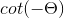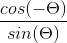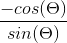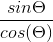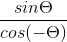Explanation:

Which of the following is equivalent to the expression:Begin by recalling the following identity: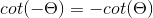Next, recall the relationship between cotangent and tangent: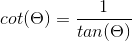As well as the relationship between tangent, sine and cosine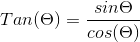So to put it all together, we can pull out the negative sign from our original expression:Next, we can rewrite our cotangent as tangent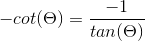Finally, we can change our tangent to sine and cosine, but because we are dealing with the reciprocal of tangent, we will need the reciprocal of our identity.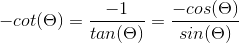This may look good on the surface, but recall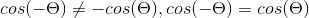Example Question #21 : Trigonometric Functions

Simplify: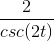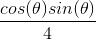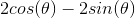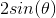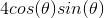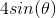Explanation:

Write the reciprocal identity for cosecant.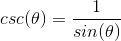Rewrite the expression and use the double angle identities for sine to simplify.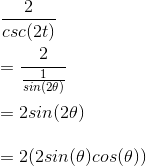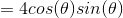Example Question #9 : Fundamental Trigonometric Identities

Determine which of the following is equivalent to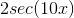.Explanation:

Rewirteusing the reciprocal identity of cosine.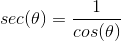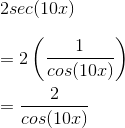Example Question #10 : Fundamental Trigonometric Identities

Which of the following is similar to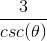?Explanation:

Write the reciprocal/ratio identity for cosecant.Replace cosecant with sine.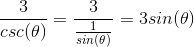Example Question #1 : Use Reciprocal And Ratio Identities

Evaluate: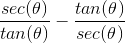Explanation:

Rewritein terms of sine and cosine.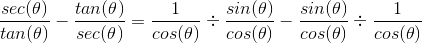Dividing fractions is the same as multiplying the numerator by the reciprocal of the denominator.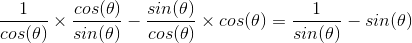Multiply the second term by sine to get a common denominator. Then after subtracting the second term from the first you can see that a Pythagorean Identity is in the numerator.

Reducing further we arrive at the final answer.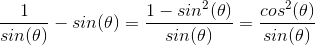Example Question #2 : Use Reciprocal And Ratio Identities

Which of the following is equal to: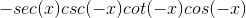Explanation:

Recall that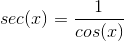, and that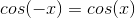Therefore: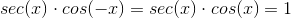Since that term is eliminated, we have left: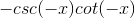Recall that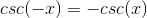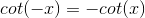Therefore: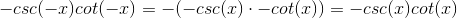All Precalculus Resources